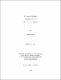## Dynamic behavior and degradation of a fuel cell system2010
##### Publisher
University of Alabama Libraries
##### Abstract

In this dissertation, the effect of dynamic operation of a Ballard Nexa 1.2kw fuel cell system is investigated. Three specific topics are considered: the first is an analysis of the dynamic behavior of the fuel cell system, the second is an evaluation and examination of fuel cell membrane degradation during dynamic operation and the last is numerical simulation to predict the transient response in the cell voltage. To enable the analysis of the fuel cell system's dynamic behavior, a simple method for analyzing the system's voltage response to a step change in load resistance is presented. A modified Randles model is used as the system model, where two resistors and two capacitors are implemented for the Warburg impedance. Using that model, the response is fitted with three exponential curves. Six independent equations corresponding to six parameters of the model can be solved using the fitted values, under a specific assumption for the initial state. The impedance is also simulated using the estimated parameters. Cyclic operation is thought to have a negative impact on fuel cell lifetime. The frequency effect of the cyclic operation on chemical degradation is investigated. After calculating each parameter value through exponential curve fitting, the dynamic behaviors of the three resistor-capacitor pairs are simulated using MATLAB Simulink®. In addition, fluoride release as the change of the frequency of cyclic operation is evaluated by measuring the concentration of fluoride ion in effluent of a fuel cell. The frequency effect on chemical degradation is explained by comparing the simulated result and the fluoride release result. Finally, a single-phase numerical model to predict the transients in voltage of a PEMFC is presented. A new approach is developed by classifying the current density by two groups; charging currents which are accumulated in the interfaces where the reaction occurs, and faradaic currents which are charge transfer currents. The successive change of the activation overpotential is calculated by using the charging currents and the element law for an ideal linear capacitor, and then the transient voltage response to a step load change is shown in results.

##### Description
Electronic Thesis or Dissertation
##### Keywords
Mechanical engineering, Computer engineering